# More Sudoku Tips: Advanced Ghosting, RC Counting, Tagging & Line Checking

by Kevin Rush (guest author)

Editor's Note: The Sudoku tips on this page are Part 3 of a three-part series of Sudoku instructions by Kevin Rush.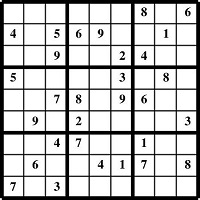This page goes further in depth with the advanced Sudoku techniques of Ghosting, RC Counting, Tagging, and Line Checking. Unless you are already an expert Sudoku player, you may want to work through the Sudoku instructions in Part 1 and Part 2 before tackling this page.

Part 1 starts Sudoku instruction from scratch. There Kevin explains the rules of Sudoku and uses a sample Easy puzzle to illustrate the two basic techniques you need to use when solving Sudoku puzzles of any difficulty, namely: "Check Each Number 1 Through 9" and "Check for One Missing Number in Each Row, Column, and Quadrant".

In Part 2, Kevin uses a Medium Sudoku sample puzzle to introduce the techniques of Ghosting, RC Counting, Tagging, and Line Checking. You'll need to understand those methods to be able to solve Medium, Hard, Very Hard, and "insane" Sudoku puzzles.

Kevin's Sudoku Instruction Pages:
Part 1: Easy Sudoku
Part 2: Medium Sudoku

When you are ready to put your Sudoku skills to the test, print out some of the 100 free Sudoku puzzles on this site or play Sudoku free online.

## Sudoku Tips: Solving a Hard Puzzle

Welcome to Part 3, the third and final part of my Sudoku Instructions. On this page, we will cover more situations using the Ghosting, RC Counting, Tagging, and Line Checking techniques we learned in Part 2.

Below is the sample puzzle we will be solving. Grab a pencil, and print the Hard Sudoku Sample (page 3) of the Sudoku Puzzle Samples document so you can follow along and fill in the puzzle as we go.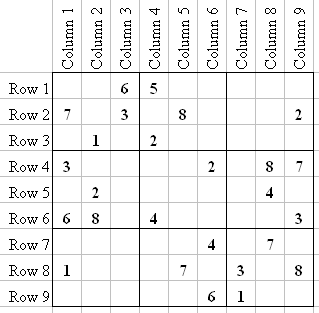By now you should be very comfortable finding Ghost numbers, RC Counting, Tagging, and Line Checking, so I will only give you the Quadrant and Square number that a number goes into, i.e. Q3-4=1. That notation means that in Quadrant 3, the entry in Square 4 is a 1.

Let's just start with the puzzle, and it will make more sense: Q1-1=2 and Q9-9=4.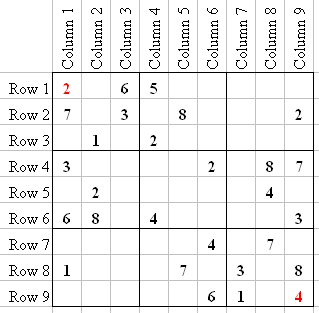Now, Line Check C1, and you'll see that Q1-7=4 (as we learned in Part 2, because every row except R3 that intersects C1 either has a number in it already in C1, or there is a 4 elsewhere in the row).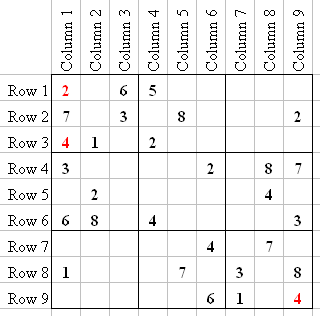Next, Q1-9=8, Q1-5=5 and Q1-2=9: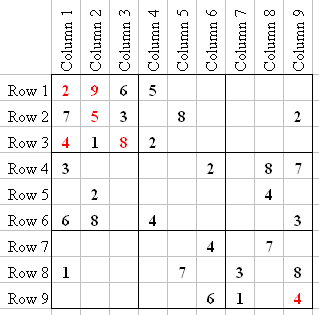In Q2 you will see the "Ghost" 7. I have highlighted it in green: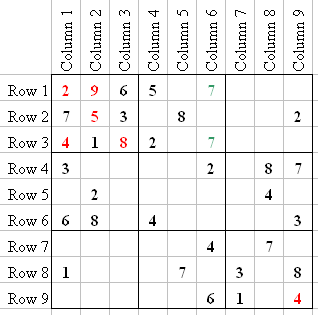Because the two Ghost 7's are both in C6, that makes Q5-4=7, which leads to Q4-9=7, and then Q7-8=7.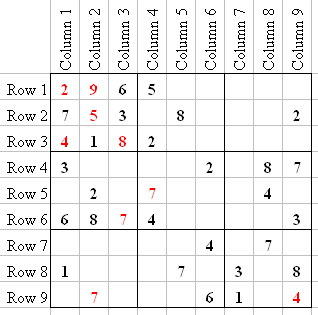Continuing next by checking numbers 1 through 9, we see that Q3-1=8 and Q5-6=8.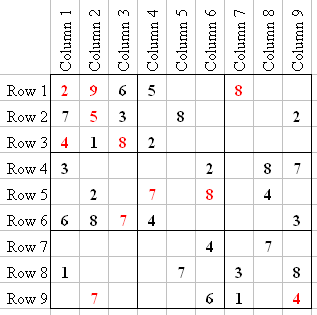Q3-7=7 and Q2-3=7. The sevens are now done.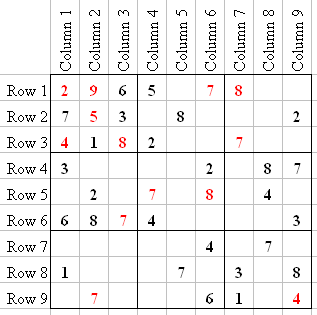Since there is only one 9 in the puzzle, there are no clear locations so let's start checking 1 through 9 again. The first number we come to that has a clear location is 3: Q5-5=3, Q2-9=3, Q3-2=3, Q7-2=3, and the last 3 at Q8-7=3.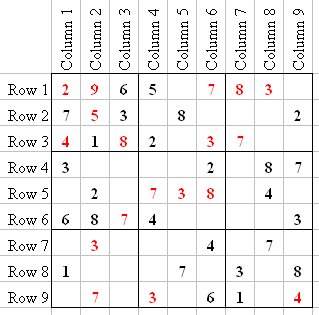Checking number 4, we see Q2-2=4 which means R1 is missing a 1 at Q3-3. Q3-4=4, Q6-7=1, Q6-6=2, and Q8-1=8; making Q7-7=8. Eights are done.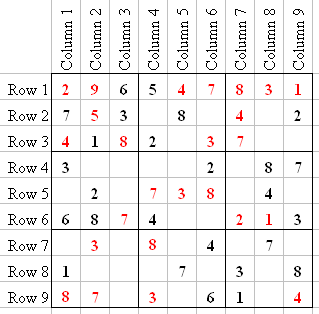An important Sudoku tip is to notice when to stop checking 1 through 9. We are in that situation now. It is easier to just look for Ghost numbers, Line Check, RC Count, or start to Tag numbers.

For example, after entering the 1 in Q6, we now have a Ghost 1 in Q5 (at Q5-1/Q5-2), which makes Q4-6=1. Line Checking C6 also shows us that Q2-6=1 (because there is a 1 in R6 and R8), and we can Tag 5 and 9 in C6, as well as 6 and 9 in Q2.

Remember when I said that in Hard puzzles you might have to Tag three or more numbers in a Quadrant? Well, now you see that we have that situation in R3, with 5, 6, and 9. We could also enter 5, 6, and 9 in the rest of C9, but that would just make the puzzle look cluttered.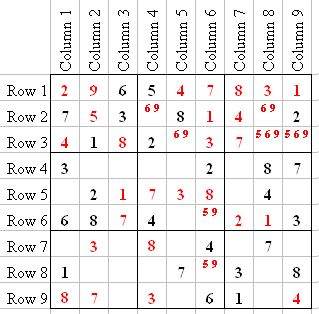Let's Line Check C2. We see that Q7-5=6, because of the 6 in Q4, so Q4-2=4.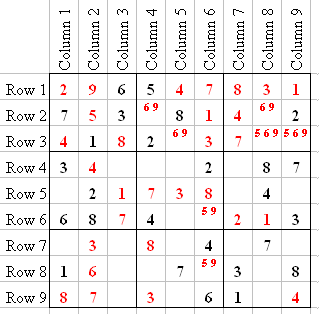Another useful Sudoku tip: I suggest that since you're just learning to solve a Hard puzzle that you always Tag squares when you see them (start with no more than three in a square, though) to help you keep track of which numbers need to go where.

The more Sudoku puzzles you solve, the less you will have to use the Tag technique. You will just start to remember them. Right now I will Tag all the rest of the squares in the puzzle that have no more than three numbers that can go into them.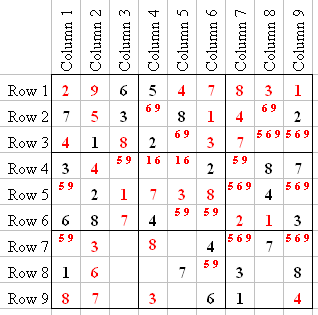Look at R7. This will take a little practice, but you will learn to notice this. R7 has two squares that aren't Tagged. The reason is that since 5, 6, and 9 are already Tagged in R7, they can't go in the last two squares of R7 (that is, those three Tagged numbers, being in three squares already, can't go into more than three). The other two numbers missing are 1 and 2. C3 already has a 1, so Q7-3 is a 2, and so Q8-2=1.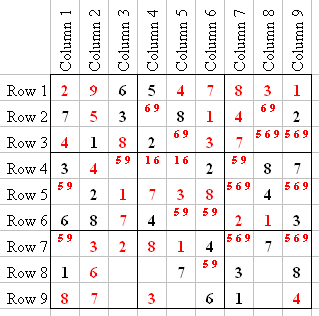Line Check C3, shows Q7-6=4, so Q7-9 is Tagged with 5 and 9. When we entered the 1 in Q8-2, that made the Tag in Q5-2=6 and Q5-1=1. Therefore, Q2-8=9, Q2-4=6, and Q3-5=9, so we can remove the 9 from Q3-8 and Q3-9 in Q3. After removing Tags and performing another round of Line Checks, we end up with this puzzle: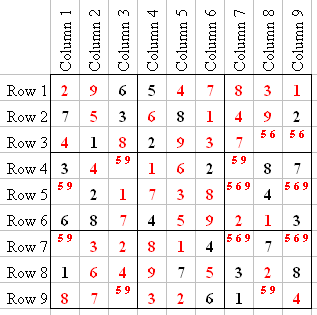Look at C8. You see we have a "5 6" Tag in Q3, and a "5 9" Tag in Q9. Well, we already have a 9 in C8 at Q3-5, so Q9-8=5, Q7-9=9, Q9-1=5, Q4-4=9, Q4-3=6, Q6-1=9, Q9-1=6, Q9-3=9, Q6-4=5, Q6-6=6, Q3-9=5, and the puzzle is finished.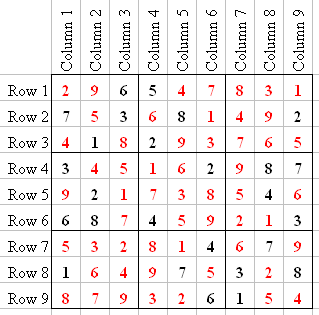This is the end of my three-part Sudoku instruction guide. Of course, what you need to do next is practice, practice, and practice some more. Print out and solve one or two Sudoku puzzles a day (or solve them online) using the Sudoku tips and tricks I've shown you, and before you know it you'll be a Sudoku pro!

~ Kevin Rush

Published: 04/10/2020
Last Updated: 06/11/2020

Free Online Brain Games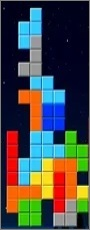No sign-up or log-in needed. Just go to a game page and start playing!Featured PostCopyscape.com